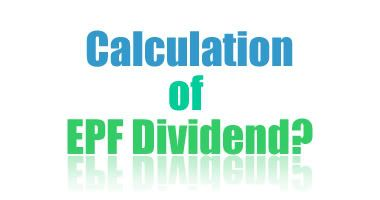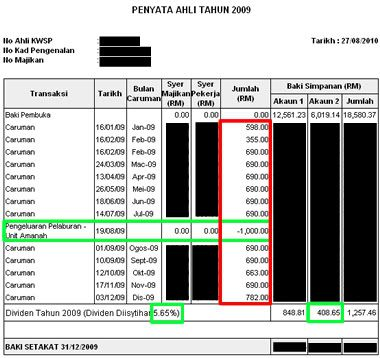# How To Calculate Your EPF Dividend?

As everybody known, the Employees Provident Fund (EPF) declared 5.65% of dividend for the financial year 2009. In order to be smart and calculative in personal finance matters, understanding the calculation of EPF dividend is an essential part of the learning process.EPF’s dividend calculation is not that straight forward. I’m sure still many of you do not know how to calculate EPF dividend. Most of the people will take the total contribution of that particular account and just multiply with 5.65% dividend. If you’re one of them, then this is a good chance for you to learn from mistake.

The following is my calculation of the dividend:i. Take your balance brought forward from year 2008 and multiply that with the dividend rate which is 5.65% directly. So, whatever you have left in year 2008 will enjoy full interest payment this year.

Balance of the savings at 1st January (EPF Account 2)
= RM 6019.14 x 5.65%
= RM 340.08

ii. Then, for monthly contribution of the year 2009, there’ll have different formula for each month:

Month’s contribution (RM) x Dividend rate (%) x (12-n*)/12

n = The number corresponding with the month,
i.e. January = 1, February = 2, etc.

*Assume that we calculate the EPF Account II dividend now, so it’s 30% of your total contribution of particular month

January, RM 179.4 (30% of RM598) x 5.65% x 11 / 12
February, RM 313.5 (30% of RM1045) x 5.65% x 10 / 12
March, RM 207 (30% of RM690) x 5.65% x 9 / 12
…….
…….

After calculated, I found that the total dividend is RM 407.9 and it’s not RM408.65. So, there may be a variation of a few cent, most probably due to KWSP’s rounding error. Don’t forget that our annual dividend on the EPF savings is calculated on daily basis. So, the another possibility would be the leap year and non-leap year case. Year 2010 only has 365 days. If not mistaken, the above formula will not take into account of leap year or non-leap year case, so you won’t get the accurate result.

Oh ya…Do you know why I take EPF Account II as my calculation? It’s because there’s withdrawal made to invest in Public Bank unit trust on 19th Aug. And, any withdrawal made for investing unit trust will affect EPF Account I dividend calculation as well.

## One thought on “How To Calculate Your EPF Dividend?”

1.iMarx says:

Dear David,

A difference between RM407.90 and RM408.65 is too LARGE for rounding errors, especially in this computing age.

Do not “believe” the formula given in the EPF website, i.e., Month’s contribution (RM) x Dividend rate (%) x (12-n*)/12 . This formula is obsolete.

See both these links (display them at your blog if you wish to) to understand how the EPF dividend is CORRECTLY and ACCURATELY calculated these days:

http://farm5.static.flickr.com/4077/4934130168_6892ae4afd_b.jpg

http://farm5.static.flickr.com/4122/4933536469_5559632de5_b.jpg

I’ve calculated for the statement you’ve displayed earlier. The figures just match PERFECTLY.

p/s: guess what, many (if not all) EPF officers don’t even know how to calculate the dividend. I’ve asked a couple of them from different branches, until I fed up and decided to decode the formula myself.